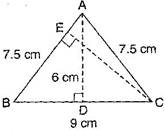### Perimeter and Area - Solutions 2

CBSE Class –VII Mathematics
NCERT Solutions
Chapter 11 Perimeter and Area (Ex. 11.2)

Question 1. Find the area of each of the following parallelograms: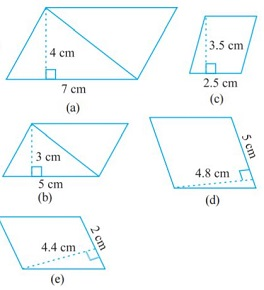Answer: We know that the area of parallelogram = base x height
(a) Here base = 7 cm and height = 4 cm
Area of parallelogram = 7 x 4 = 28 cm2
(b) Here base = 5 cm and height = 3 cm
Area of parallelogram = 5 x 3 = 15 cm2
(c) Here base = 2.5 cm and height = 3.5 cm
Area of parallelogram = 2.5 x 3.5 = 8.75 cm2
(d) Here base = 5 cm and height = 4.8 cm
Area of parallelogram = 5 x 4.8 = 24 cm2
(e) Here base = 2 cm and height = 4.4 cm
Area of parallelogram = 2 x 4.4 = 8.8 cm2

Question 2. Find the area of each of the following triangles: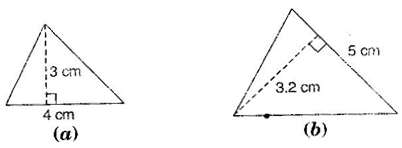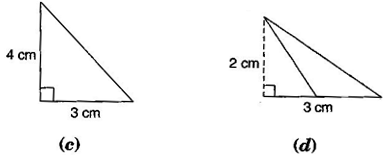Answer: We know that the area of triangle =  x base x height
(a) Here, base = 4 cm and height = 3 cm
Area of triangle =  x 4 x 3 = 6 cm2
(b) Here, base = 5 cm and height = 3.2 cm
Area of triangle =  x 5 x 3.2 = 8 cm2
(c) Here, base = 3 cm and height = 4 cm
Area of triangle =  x 3 x 4 = 6 cm2
(d) Here, base = 3 cm and height = 2 cm
Area of triangle =  x 3 x 2 = 3 cm2

Question 3. Find the missing values:
 S. No. Base Height Area of the parallelogram a. 20 cm 246 cm2 b. 15 cm 154.5 cm2 c. 8.4 cm 48.72 cm2 d. 15.6 cm 16.38 cm2
Answer: We know that the area of parallelogram = base x height
(a) Here, base = 20 cm and area = 246 cm2
Area of parallelogram = base x height
246 = 20 x height  height =  = 12.3 cm
(b) Here, height = 15 cm and area = 154.5 cm2
Area of parallelogram = base x height
154.5 = base x 15  base =  = 10.3 cm
(c) Here, height = 8.4 cm and area = 48.72 cm2
Area of parallelogram = base x height
48.72 = base x 8.4  base =  = 5.8 cm
(d) Here, base = 15.6 cm and area = 16.38 cm2
Area of parallelogram = base x height
16.38 = 15.6 x height  height =  = 1.05 cm
Thus, the missing values are:
 S. No. Base Height Area of the parallelogram a. 20 cm 12.3 cm 246 cm2 b. 10.3 cm 15 cm 154.5 cm2 c. 5.8 cm 8.4 cm 48.72 cm2 d. 15.6 cm 1.05 16.38 cm2

Question 4. Find the missing values:
 Base Height Area of triangle 15 cm --- 87 cm2 --- 31.4 mm 1256 mm2 22 cm --- 170.5 cm2
Answer: We know that the area of triangle =  x base x height
In first row, base = 15 cm and area = 87 cm2
87 =  x 15 x height  height =  = 11.6 cm
In second row, height = 31.4 mm and area = 1256 mm2
1256 =  x base x 31.4  base =  = 80 mm
In third row, base = 22 cm and area = 170.5 cm2
170.5 =  x 22 x height  height =  = 15.5 cm
Thus, the missing values are:
 Base Height Area of triangle 15 cm 11.6 cm 87 cm2 80 mm 31.4 mm 1256 mm2 22 cm 15.5 cm 170.5 cm2

Question 5. PQRS is a parallelogram. QM is the height from Q to SR and QN is the height from Q to PS. If SR = 12 cm and QM = 7.6 cm. Find:
(a) the area of the parallelogram PQRS
(b) QN, if PS = 8 cm
Answer: Given: SR = 12 cm, QM= 7.6 cm, PS = 8 cm.
(a) Area of parallelogram = base x height = 12 x 7.6 = 91.2 cm2
(b) Area of parallelogram = base x height
91.2 = 8 x QN  QN =  = 11.4 cm

Question 6. DL and BM are the heights on sides AB and AD respectively of parallelogram ABCD. If the area of the parallelogram is 1470 cm2, AB = 35 cm and AD = 49 cm, find the length of BM and DL.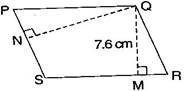Answer: Given: Area of parallelogram = 1470 cm2
Base (AB) = 35 cm and base (AD) = 49 cm
Since Area of parallelogram = base x height
1470 = 35 x DL  DL =  = 42 cm
Again, Area of parallelogram = base x height
1470 = 49 x BM  BM =  = 30 cm
Thus, the lengths of DL and BM are 42 cm and 30 cm respectively.

Question 7. ABC is right angled at A. AD is perpendicular to BC. If AB = 5 cm, BC = 13 cm and AC = 12 cm, find the area of ABC. Also, find the length of AD.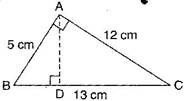Answer: In right angled triangle BAC, AB = 5 cm and AC = 12 cm
Area of triangle =  x base x height =  x AB x AC =  x 5 x 12 = 30 cm2
Now, in ABC,
Area of triangle ABC =  x BC x AD